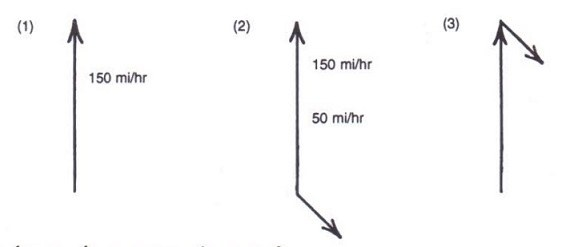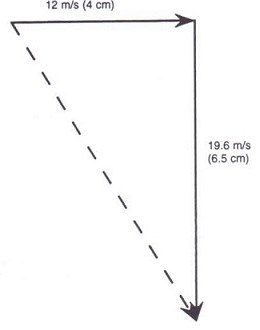# Mathematical methods of vector addition

👁 117
statistics

Mathematical methods to calculate vector addition and net force

Questions #: 3
Time: 5 minutes
Pass Score: 80.0%
Style
Mode

#### Given the below figure the net force is 220 lb, what are the mathematical steps to get the net force?

Cars collisionUse arrows to represent exerted forces
Use comparable measurement units to forces (e.g. cm)
Replace the image with an abstract figure showing cars as arrows with headings
The more the force the more the length of the arrow
Hock arrows end-to-end but keep length and direction
Draw an arrow from the tail of the long arrow to the head of the short arrow
measure the length of the new arrow and multiply by value of lb = 1 cm
POINTS (1)

#### Flying airplaneConsider an airplane flying in still air at a velocity of 150 miles per hour northward.  In part 1 of the figure below, a 3-cm arrow represents this velocity.  Now a wind starts blowing toward the south east at a speed of 50 mi/hr-quite a wind!  The second part of the figure shows this 50-mi/hr wind.  In part 3, one arrow is moved so that its tail falls on the head of the other.  Assume that the angle between the two lines is 900

Please find the results of the below statements

1. 1 cm = lb
2. The length of the wind vector in drawing (cm) =
3. Approximated net force in  (lb) =
POINTS (1)

#### Balloon dropping a sandbag (projectile motion)

A balloon is high enough that the sandbag hits the ground 2 seconds after it is released.  At that time the forward speed of the sandbag is still 12 m/s.  Its downward speed is now 19.6 m/s.This drawing uses a scale of cm to represent m/s.  The arrow that shows the total is cm long, so the total velocity is m/s.

POINTS (1)